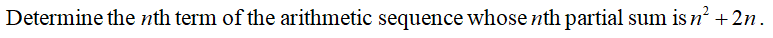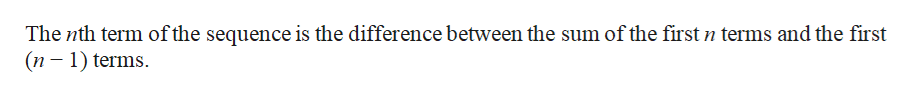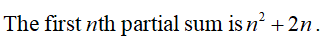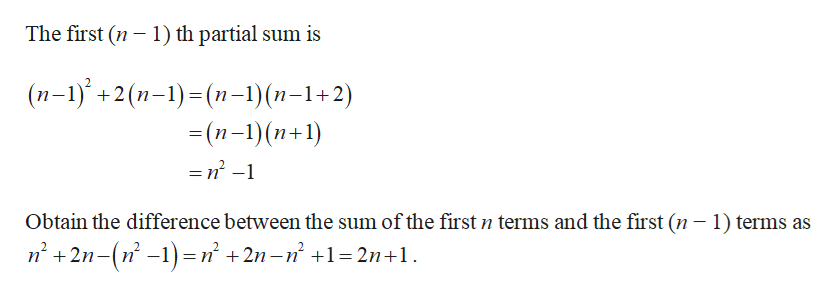# Determine the nth term of the arithmetic sequence whose nth partial sum is n2 + 2n. [Hint: The nth term of the sequence is the difference between the sum of the first n terms and the first (n − 1) terms.]

Question
1 views

Determine the nth term of the arithmetic sequence whose nth partial sum is n2 + 2n. [Hint: The nth term of the sequence is the difference between the sum of the first n terms and the first (n − 1) terms.]

check_circle

Step 1help_outlineImage TranscriptioncloseThe nth term of the sequence is the difference between the sum of the first n terms and the first (n – 1) terms. fullscreen
Step 2...help_outlineImage TranscriptioncloseThe first (n – 1) th partial sum is (п-1)* +2 (п-1)-(п-1) (n-1+2) - (п-1) (п+1) =n -1 Obtain the difference between the sum of the first n terms and the first (n – 1) terms as n' +2n-(n -1) =n² +2n–n° +1= 2n+1. fullscreen

### Want to see the full answer?

See Solution

#### Want to see this answer and more?

Solutions are written by subject experts who are available 24/7. Questions are typically answered within 1 hour.*

See Solution
*Response times may vary by subject and question.
Tagged in
MathAlgebra

### Sequence and Series